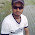### Board Exam Refresher: Machine Design (calculation)

Here are some refresher questions you may try in preparation for the upcoming board exam.

Machine Design: Strength of Materials

1. A steel rod 40 mm in diameter 800 mm long cannot extend longer than 41.5 mm. Find the maximum allowable load it can carry in Newtons. E for steel is 200 GPa.
A. 471240
B. 600000
C. 188495
D. 712440

2. A simply supported beam 3m long has a load of 3KN 1 m from the left support. Calculate the maximum moment.

A. 2.25 kN-m
B. 2.0 kN-m
C. 4.5 kN-m
D. 6.0 kN-m

3. Calculate the maximum deflection of a 12 m beam with fixed ends if the load applied on it is 3KN located at its center. (I=4,200,000 E=200 GPa).

A. 128.6 mm
B. 32.14 mm
C. 0.17 mm
D. 0.27 mm

4. A 40mm short shaft has is rotating at 300RPM. Calculate the power capacity.
For short shaft: (PME Code)
A. 30.83 kW
B. 23 kW
C. 16.34 kW
D. 10.93 kW

5. Calculate the wall thickness required for a 1m spherical thin walled vessel carrying 30Mpa of fluid pressure. The maximum allowable stress is 150Mpa.
A. 100mm
B. 200 mm
C. 150 mm
D. 50 mm

6. A 33 mm long single leaf spring is carrying a load of 50 grams 3 mm from its tip. If the material used in making the spring is a 0.3 mm sheet metal, calculate the required width if the allowable stress is not to exceed 250 Mpa.

A. 4.12 mm
B. 0.71 mm
C. 3.92 mm
D. 1.18 mm

7. A compression spring has the following data:
Wire diameter: 0.5 mm
Solid height: 5mm
OD: 4 mm
Calculate the Wahl’s factor.
A. 1.213
B. 1.184
C. 1.145
D. 1.162

8. A spring has a Wahl’s factor of 1.212, a free length of 8 mm, and an index of 7 calculate the curvature factor.

A. 1.125
B. 1.051
C. 0.962
D. 1.131

9. A 20 mm diameter, 700 cm long rod elongates 2 mm at a load of 15KN. Calculate the modulus of elasticity of the material used.

A. 167 Gpa
B. 668 GPa
C. 743 GPa
D. 334 GPa

10. Calculate the force required to punch a 10 mm diameter hole into a 2 mm thick sheet metal with the these properties: Sy=155 Mpa Su=300 Mpa

A. 11.31 kN
B. 9.74 kN
C. 18.85 kN
D. 14.14 kN

Answers will be provided next week.

1.It is nice information about GPA Calculator. i think its really helpful for all of modern student.

### BOARD EXAM TIP: Which Review Center?

First of all, I would like to apologize for a long time I haven't posted anything but introduction. The reason for that is I'm busy optimizing the design of this site. I'm hoping that the make over is good. Anyway, I would like to dedicate this article to those who are going to take/retake the board exam this coming October. My sincere wish that you bring out the best among yourselves and may the deserving ones pass the exam.

Going back to the One-Million-Peso worth question: "Which review center should I enroll?", I would like to answer that in the most unbiased way I can. Of course, I'm only human and I had also my preferences but I will try my best to hide it.

To start with I will enumerate some of the prominent review centers that offer board exam reviews for mechanical engineering in the Philippines.

1. Alcorcon (Cebu & Manila)
2. Excel (Cebu & Manila)
3. Linx Engineering (Manila)
4. MERIT (Manila)
5. PRIME (Cebu)
6. RLB's CDTC (Manila)
I'…

### PAST MECHANICAL BOARD EXAM QUESTION: Machine Design Set-1 (solutions and answers)

The following are the solutions and answers to MODIFIED PAST MECHANICAL BOARD EXAM QUESTION: Machine Design Set-1 posted on this blog last Saturday, November 14, 2009.

Again, I want to stress out that most of the questions here are modified from its original form for the purpose of improving the question.

Past Mechanical Engineering Board Exam Question Number 1
A 4 inches diameter short shaft carrying 2 pulleys close to the bearings transmits how much horsepower if the shaft makes 120 rpm.

A.200HP               B. 199 HP

C.198 HP              D. 202 HP

P = (D3N )/38 -- for mula for short shafts from PSME code

P = (4)3(120)/38

P= 202 HP

Past Mechanical Engineering Board Exam Question Number 2
An instrument that measures the hardness of the work piece in terms of elasticity.

a. Durometer

b. Scleroscope

c. Mohs's Scale

d. Brinell Tester

Source: Machinery’s Handbook

### SOLUTION TO MECHANICAL BOARD EXAM PROBLEMS: Power Set-1

Here are the solutions to board problems given at Power Subject Set-1. If you do not understand anything, feel free to write a comment voicing out your concern. I'm still looking for better ways to present my solution. I still don't know how to present equations and mathematical symbols with blogger. Please bear with me.

1. An automobile tire is inflated to 32psig pressure at 50 degree F. After being driven, the temperature rises to 75 degree F. Determine the final gage pressure assuming the volume remains constant. (Electrical Engineering Board Exam Problem)

Solution:
Since volume is constant, use Charles’ Law on constant volume processes.
P1/T1=P2/T2
Note: P and T should be absolute.

(32+14.7)/ (50+460) =P2/ (75+460)

P2=48.99 psia

Converting back to gage pressure,

P2g=48.99-14.7

P2g=34.29 psig

2. Four hundred cubic centimeters of gas at a pressure of 740 mmHg absolute and temperature of 18 degree C undergoes a process until the pressure and temperature becomes 760 mmHg abso…